# Community

Blog Events Webinars Tutorials Forum
×
Community Blog Demystifying Common Machine Learning Terms

# Demystifying Common Machine Learning Terms

This article gives an overview of the common concepts used in machine learning and artificial intelligences.

On a higher level, machine learning is a straightforward process. A computer (or machine) receives input (data) and analyzes (algorithm) the data to create or improve data models. These data models are then used to describe or predict behaviors in real-world applications. However, effectively implementing data models and algorithms is a huge challenge.

There are many machine learning algorithms available, but unlike human learning, these algorithms are typically confined to a particular application. Algorithms are important because they are used to construct and evaluate data models. If an evaluation result indicates that the performance meets needs, the data model is used to test other data. If the performance does not meet requirements, algorithms are adjusted to set up and evaluate another model. This process is repeated until satisfying experiences are obtained to process other data.

Technologies and methods of machine learning get successfully applied to many fields, such Taobao's recommendation system, anti-fraud solutions in Ant Financial, and voice recognition and natural language processing of TMall Genie. In this article, we will take a closer look at some of the commonly used terms in machine learning.

## Classification of Machine Learning

### Supervised Learning

In supervised learning, a model is learned from the given training data and gets subsequently used for prediction. The prediction result is compared with the actual result, and then the prediction model is continuously adjusted until an expected accuracy rate gets achieved. Contrary to popular belief, the term "supervised" does not mean manual intervention during the learning process. Most of this so-called "supervised" learning are done through mathematical modelling of existing data.

Standard supervised learning algorithms include regression analysis and statistical classification. Supervised learning often gets used for training the neural network and decision tree. These algorithms are highly dependent on predefined classification systems, such as spam and news content classification.

### Unsupervised Learning

The training set in unsupervised learning does not have any manually labeled result. The learning model is some internal structures used to deduce data. Common application scenarios include association rule learning and clustering.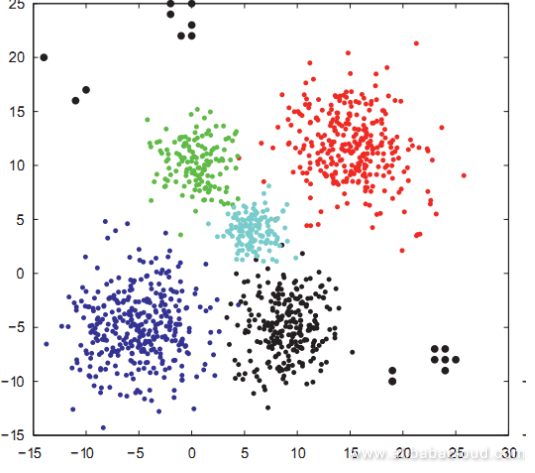Unsupervised learning aims to locate the approximate point in the training data, instead of maximizing the utility function. Clustering can often find the classification that matches well with assumptions.

### Semi-Supervised Learning

Semi-supervised learning is somewhere between supervised learning and unsupervised learning. Its major concern is how to use a few labeled samples and a large number of unlabeled samples for training and classification. The learning algorithms attempt to set up a model for unlabeled data and then predict the labeled data. Examples of semi-supervised learning include graph inference and Laplacian SVM.

## Common Machine Learning Algorithms

### Regression Algorithms

Regression algorithms include ordinary least square, logistic regression, stepwise regression, multivariate adaptive regression splines, and locally estimated scatterplot smoothing.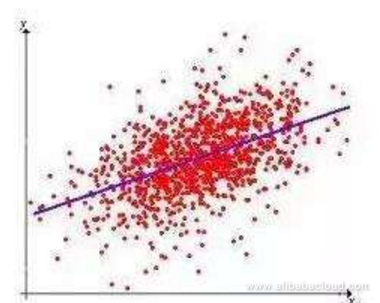### Instance-Based Algorithms

These algorithms are often called "Winners Take All" learning. They are commonly used to set up models for countermeasures. This type of model usually selects a batch of sample data and compares new data with sample data based on some approximations to find the optimal match.

### Decision Tree Learning

Based on data attributes, the tree structure is used to set up a decision-making model. Decision tree learning is often used to resolve the classification and regression problems.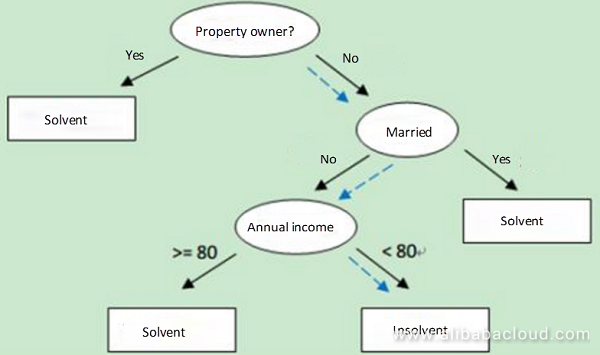### Bayesian Learning

Bayesian learning is mainly used to resolve the classification and regression problems. An example of Bayesian learning is the naive Bayesian algorithm.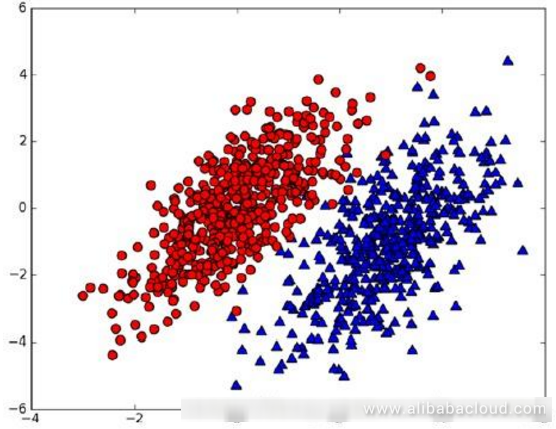### Cluster and Classification Algorithms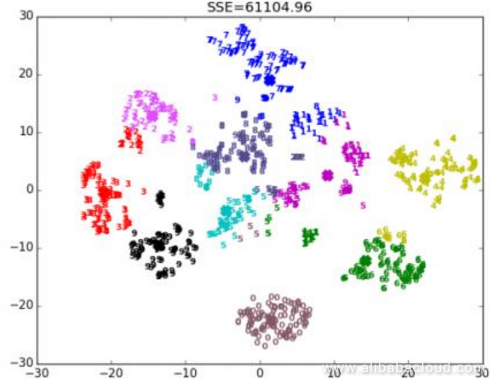Cluster and classification are two types of algorithms that are often get used in machine learning. Clustering divides data into different sets, whereas classification predicts the class of new data.

What is Clustering?
In clustering, data objects are divided into different groups or subnets using a static classification method. The objective of clustering is that objects in the same cluster have a similar attributes, whereas objects in different clusters differ a lot from each other. Clustering analysis can be considered an unsupervised learning technology because these attributes are not pre-defined in the algorithm.

What is Classification?
Classification is an important machine learning and data mining technology. The purpose of classification is to construct a classification function or model (often called classifier) based on characteristics of data sets. This model can map samples of unknown classes to technology in a specific class.

Classification is a type of supervised learning as the classes are pre-defined. The process of building a classification model is generally divided into two phases: training and test. Before model construction, data sets are divided into training data sets and test data sets at random. Training data sets are used to construct a classification model. Then, the test data sets are used to evaluate the classification accuracy of the model. If the model accuracy is acceptable, this model is used to classify other data tuples. Generally, the cost of the test phase is far lower than that of the training phase.

## Conclusion

Machine learning, although conceptually simple, is challenging to be implemented effectively. Most machine learning methods can be categorized into supervised learning and unsupervised learning. Typically, unsupervised is used for analyzing data or generating new insights to help enterprises better understand user behavior. Supervised learning on the other hand, is more effective for creating effective prediction algorithms by analyzing historical data.

0 0 0
Share on

# Related Products

• ##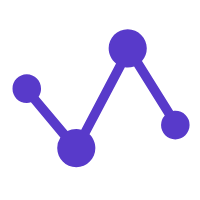MaxCompute

Conduct large-scale data warehousing with MaxCompute

• ##E-MapReduce Service

A Big Data service that uses Apache Hadoop and Spark to process and analyze data

• ##DataWorks

A secure environment for offline data development, with powerful Open APIs, to create an ecosystem for redevelopment.